by: babak babaabasi

## R If … Else

### Conditions and If Statements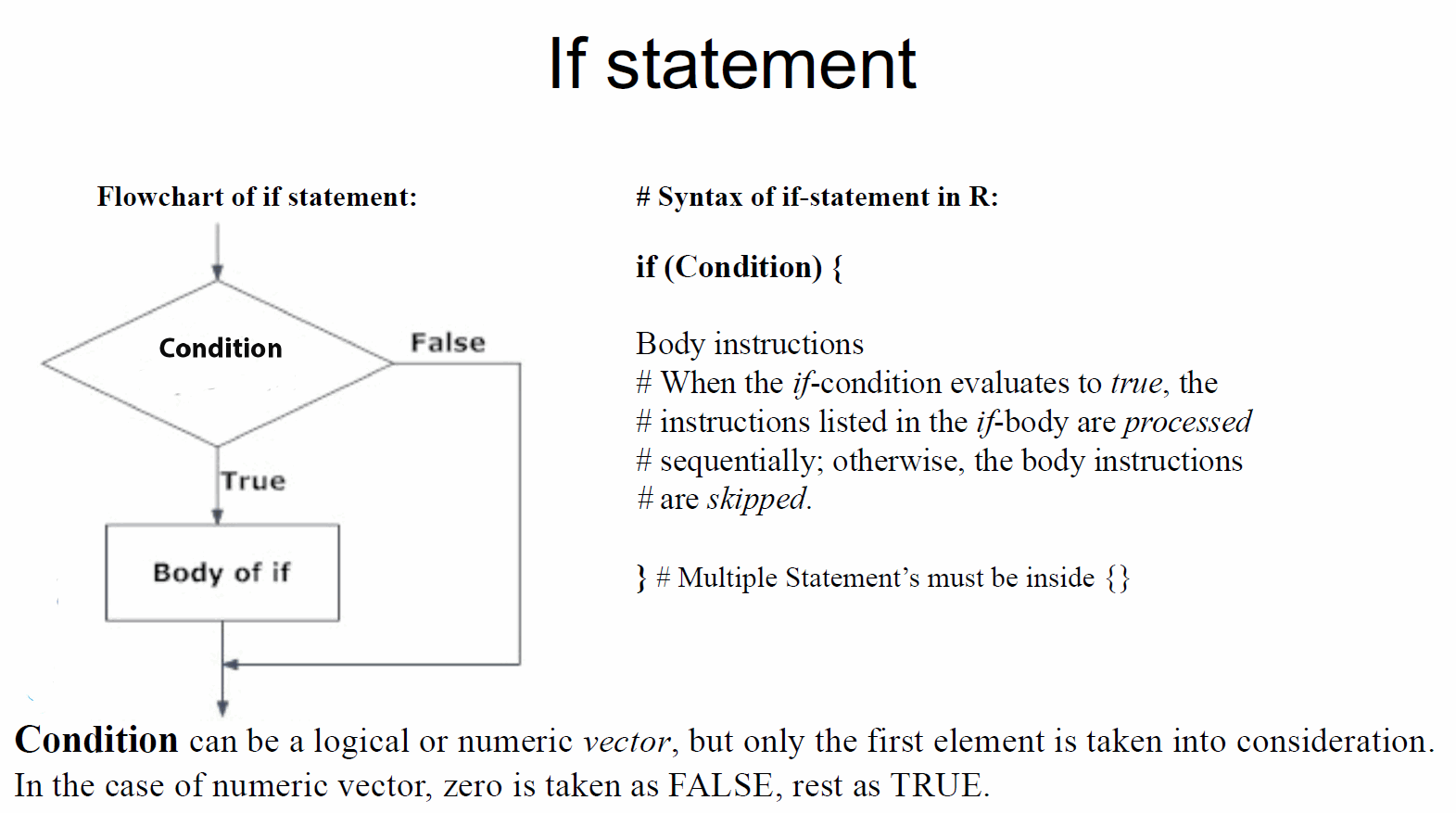x = readline(prompt="Enter an integer number: ")

if(x>3) print("Positive")
if(x<7) print("negative")

Write the R script that take two integers as input and print out the larger number.

A = as.numeric(readline(prompt="Enter first numbera: "))
B = as.numeric(readline(prompt="Enter second number: "))
if(A > B) print(A)
if(B > A) print(B)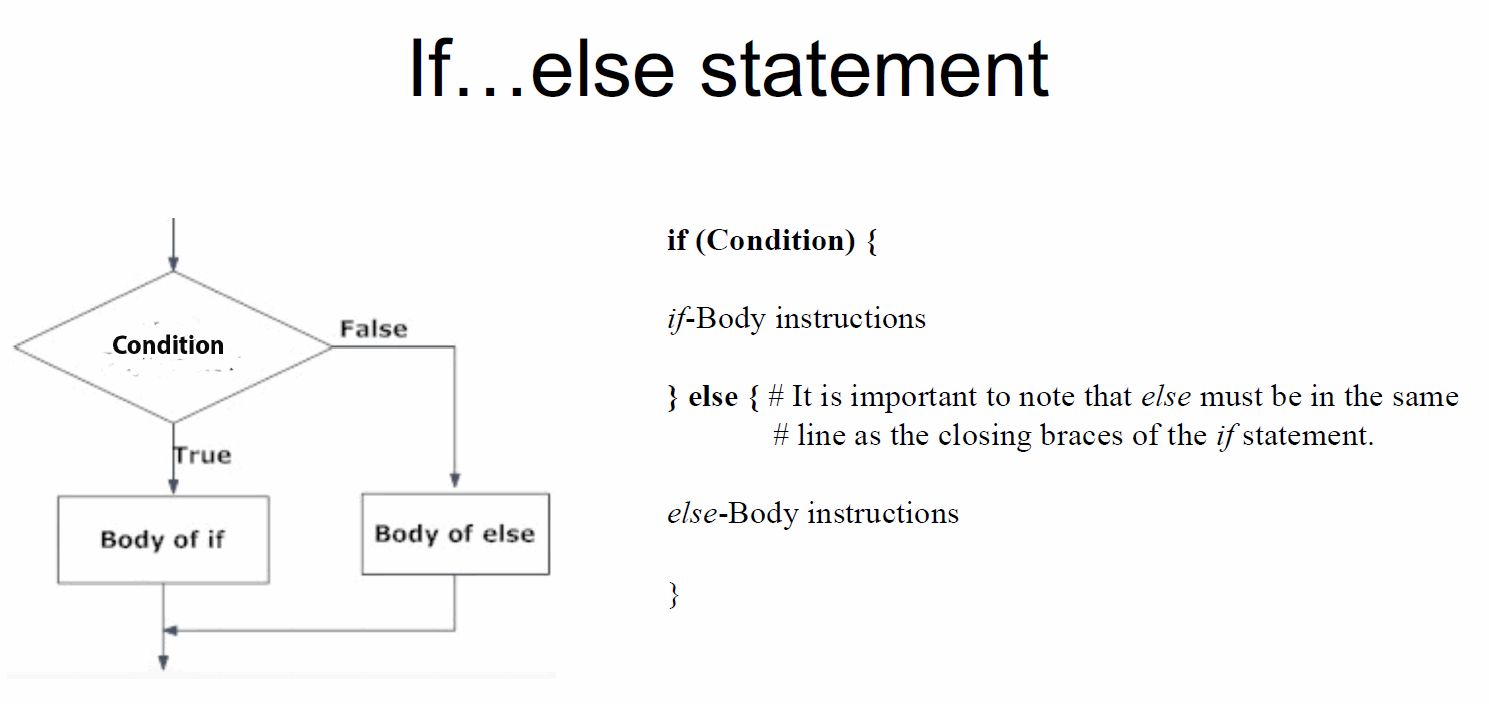x = as.integer(readline(prompt="Enter an integer number: "))
if(x>0) print("Positive") else print("Negative")

You can also run a condition in an if statement, which you will learn much more about in the if..else chapter.

a = 200
b = 33

if (b > a) {
print ("b is greater than a")
} else {
print("b is not greater than a")
} 
if (b > a) {
(b*4)
} else {
(b*2)
}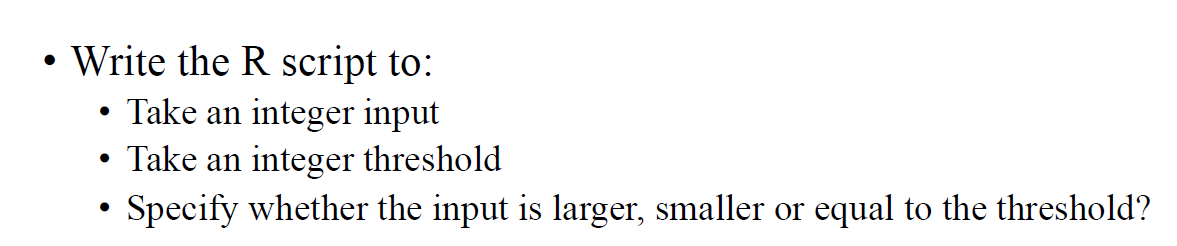A = as.integer(readline(prompt="Enter an integer number: "))
B = as.integer(readline(prompt="Enter an integer threshold: "))
if(A > B){
print(paste(A, "is greater than threshold ", B), quote=F)
} else if (A < B){
print(paste(A, "is less than threshold ", B), quote=F)
} else {
print (paste(A, "is equal to threshold ", B), quote=F)
}
a <- 200
b <- 33

if (b > a) {
print("b is greater than a")
} else if (a == b) {
print("a and b are equal")
} else {
print("a is greater than b")
} 

### Nested If Statements

x <- 44

if (x > 10) {
print("Above ten")
if (x > 20) {
print("and also above 20!")
} else {
print("but not above 20.")
}
} else {
print("below 10.")
} 

## AND

The & symbol (and) is a logical operator, and is used to combine conditional statements:

a <- 200
b <- 33
c <- 500

if (a > b & c > a){
print("Both conditions are true")
}

## OR

The | symbol (or) is a logical operator, and is used to combine conditional statements:

a <- 200
b <- 33
c <- 500

if (a > b | a > c){
print("At least one of the conditions is true")
} 

##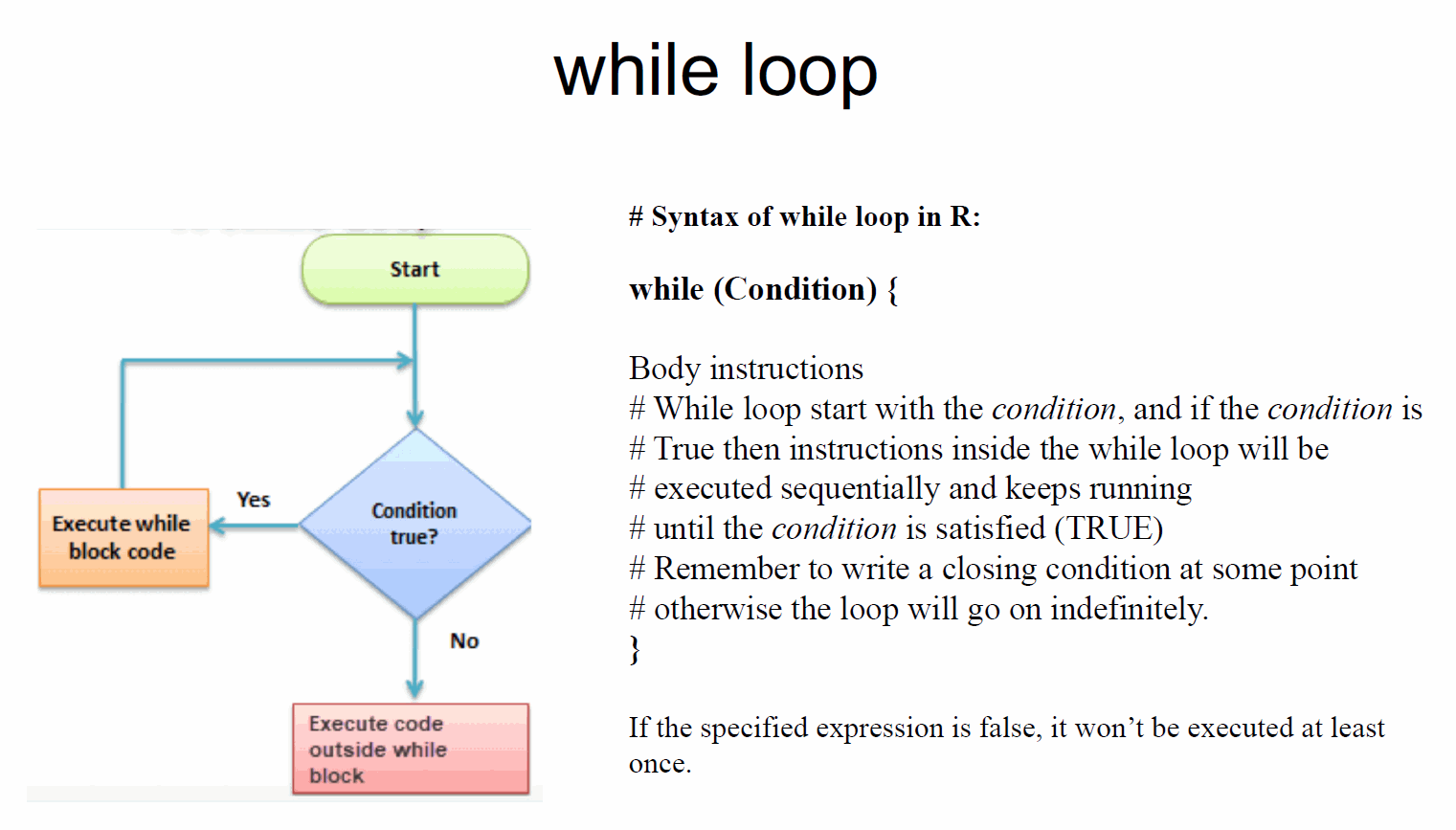i = 1
while (i < 6) {
print(i)
i = i+1
}
a=c(45,67,89,90,56,44,78,34)
i = 1
l=length(a)
f=0
while (i <= l) {
f=a[i]+f
i = i+1
m=f/l
print(m)
}
# R program to illustrate
# application of while loop

# assigning value to the variable
# whose factorial will be calculated
n < - 5

# assigning the factorial variable
# and iteration variable to 1
factorial < - 1
i < - 1

# using while loop
while (i <= n)
{

# multiplying the factorial variable
# with the iteration variable
factorial = factorial * i

# incrementing the iteration variable
i = i + 1
}

# displaying the factorial
print(factorial)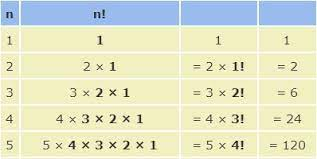n = 5
factorial = 1
i = 1

while (i <= n){
factorial = factorial * i
i = i + 1
}

print(factorial)

##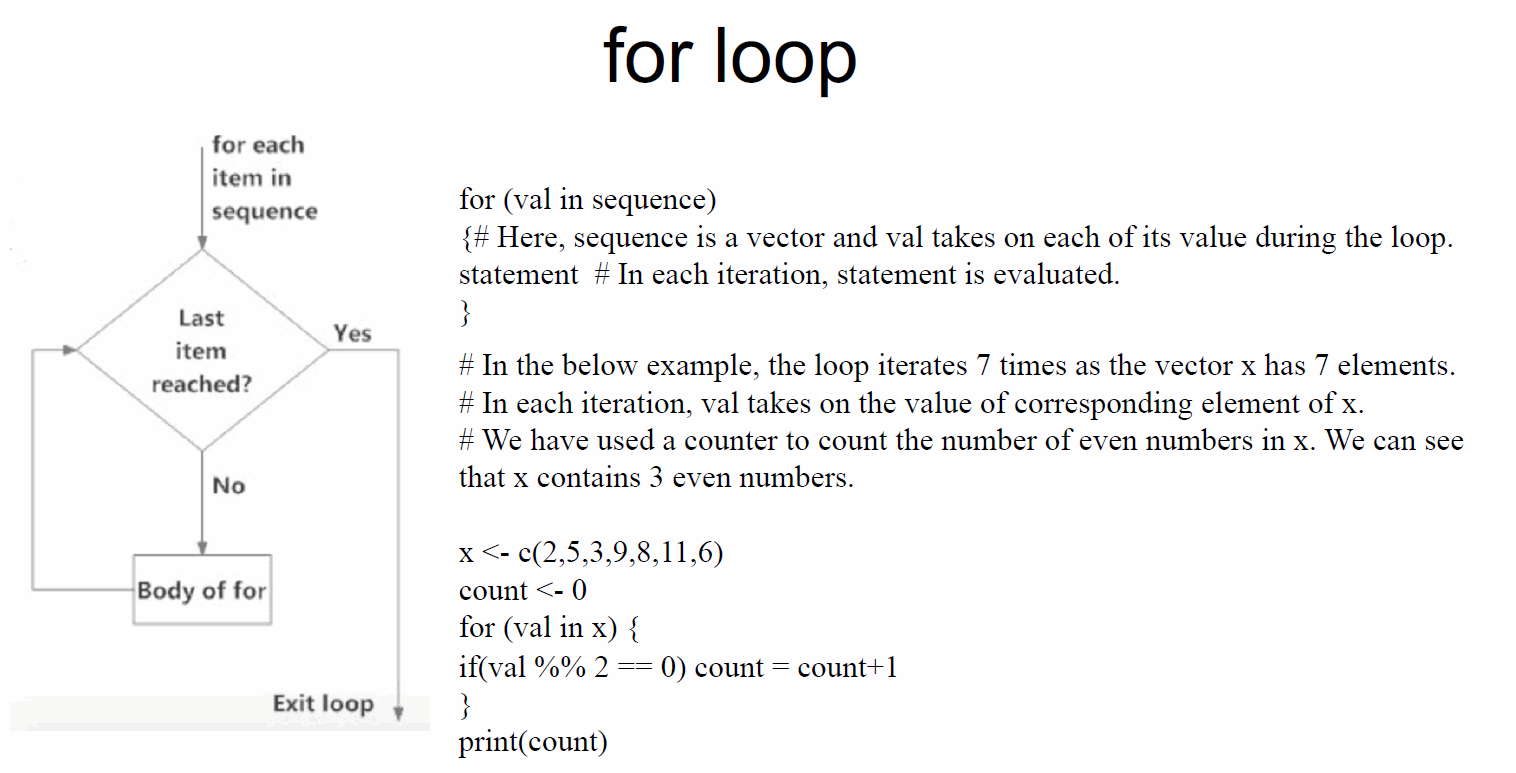for (val in sequence)
{
statement
}
f = c(2,5,3,9,8,11,6,11,12,20)
count = 0
for (val in f) {
if(val %% 2 == 0)
count = count+1
}
print(count)

factorial by for

num =6
factorial = 1
for(i in 1:num) {
factorial = factorial * i
}
print(paste("The factorial of", num ,"is",factorial))

factorial by for

# take input from the user
num = as.integer(readline(prompt="Enter a number: "))
factorial = 1
# check is the number is negative, positive or zero
if(num < 0) {
print("Sorry, factorial does not exist for negative numbers")
} else if(num == 0) {
print("The factorial of 0 is 1")
} else {
for(i in 1:num) {
factorial = factorial * i
}
print(paste("The factorial of", num ,"is",factorial))
}